# Electronics and Communication Engineering - Exam Questions Papers

36.

The question below consists of a pair of related words followed by four pairs of words. Select the pair that best expresses the relation in the original pair.
Unemployed: Worker

 A. fallow : land B. unaware : sleeper C. wit : jester D. renovated : house

Explanation:

A worker who is inactive or not working is termed as unemployed, similarly land which is inactive or not in use is called Fallow.

37.

An FM transmitter delivers 80W to a load of 30 W when no modulation is present. The carrier is now frequency modulated by a single sinusoidal signal and the peak frequency deviation is so adjusted to make the amplitude of the second sideband is zero in the given output.
J0(0, 0) = 1,
J0(2, 4) = 0,
J0(3.8) = - 0.4,
J0(5.1)= - 0.1
J1(2, 4) = 0.52,
J1((3.8) = 0,
J1(5.1) = - 0.33,
J2(2.4) = 0.1
J2(3.8)= 0.41,
J2(5.1) = 0
Power in the first order sidebands is :

 A. 5.12 W B. 21.78 W C. 2.56 W D. 16.81 W

Explanation:

Power in first order side bands = 2(J1(β))2 x 100 = 21.78 W.

38.

For a binary symmetric channel, the error probability Pc(0) = Pc(1) =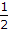and errors are statistically independent. The channel capacity for a signalling speed 2 bits/second.

 A. 2 bits/sec B. 1.69 bits/sec C. 4 bits/sec D. 1 bit/sec

Explanation:

C = r . H

r = 2 bits/second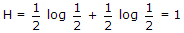C = 2 bits/second.

39.

Figure below shows D type Flip-Flops connected as shift register, then outputs QD, QC, QB, QA are given by: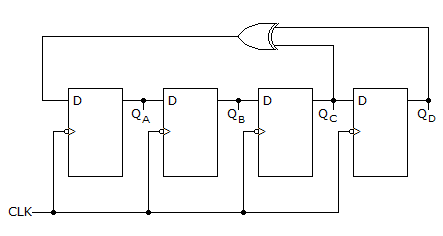1.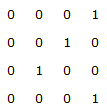2.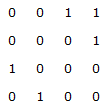3.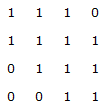A. 1 and 2 only B. 2 and 3 only C. 1, 2 and 3 D. none of these

Explanation:

Here DA = QD ⊕ QC; DB = QA; DC = QB;

DD = QC

For the given input string, outputs are QD, QC, QB, QA.

Therefore right most bit is QA and left most bit is QD.

Now we determine the string sequence according to the above mentioned equations.

(i)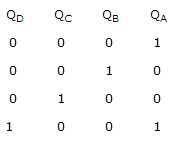(ii)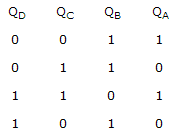(iii)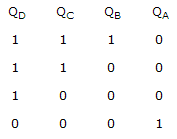40.

To realize following function 'f',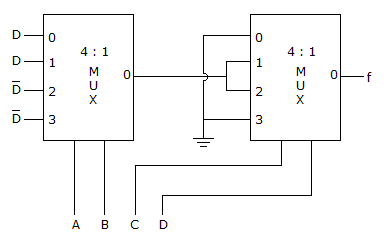How many minimum number of 2 input NAND gates are required?

 A. 3 B. 6 C. 4 D. 10

Explanation:

f1 = A B D + A B C + A B D + A B D = A D + A D = A ⊕ D

f = C D(A D + A D) + C D(A D + A D + C D A + C D A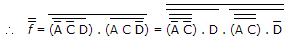Min number of 2 input NAND gate = 10.

#### Current Affairs 2022

Interview Questions and Answers